In :
%matplotlib inline
import sqlite3
import os
import pandas as pd
import numpy as np
import pyarrow
import matplotlib.pyplot as plt
import seaborn as sns
import matplotlib.gridspec as gridspec
from matplotlib.pyplot import figure
import random
import warnings
warnings.filterwarnings("ignore")
In :
# CONFIGURE THE BIGQUERY SETTINGS
BIGQUERY_PROJECT = 'ironhacks-covid19-data'
BIGQUERY_KEYPATH = 'service-account.json'

bigquery_client = bigquery.Client(project=BIGQUERY_PROJECT)

conn = sqlite3.connect("Chinook_Sqlite.sqlite")
cursor = conn.cursor()

In :
query = """
SELECT *
FROM ironhacks_covid19_competition.weekly_patterns
"""
query_job = bigquery_client.query(query)
fd = query_job.to_dataframe()

In :
prediction = pd.read_csv("avg.csv")
prediction = prediction.rename({'avg': 'raw_visit_counts'}, axis=1)
prediction['week_number'] = 44
# df.merge(prediction, left_on=['poi_id','week_number'])
df = pd.concat([fd, prediction]).sort_values(by=['poi_id','week_number']).ffill()
# fd.drop('raw_visit_counts', 1).fillna(fd.median())


# Overall Visulizations¶

Let's look at some data in the big picture and find some obvious trend in the overall data.

### Weekly Visits Trend¶

The graph below is a graph for number of total visits to all places for each week. We can see that the total number of visits made started to incrase exponentially starting form week 30 all the way to week 33 and drops after that. I looked up the data and week 33 is the week that Purdue starts. My explaination for the peak is that student started to come back since week 30 and went to places because the school hasn't started yet and most students were on campus around week 33. After the school has started, the students had less time to go out due to school work.

In :
total = df.groupby('week_number')['raw_visit_counts'].sum()
plt.plot(total[:-1].index, total[:-1].values)
plt.plot(total.index[-1], total.iloc[-1], marker="o", color='r', lw=20)
plt.text(42, 160000, "Prediction")

plt.annotate("Purdue Fall Semester Starts", xy = (33, 201000), xytext = (28, 210000),
bbox=dict(boxstyle="round",  fc=(1.0, 0.7, 0.7), ec=(1., .5, .5)),
arrowprops=dict(arrowstyle="wedge,tail_width=1.", connectionstyle="arc3,rad=-0.7", fc=(1.0, 0.7, 0.7), ec=(1., .5, .5),relpos=(0.2, 0.8)))
plt.xlabel('Week Number')
plt.ylabel('Total Raw Visit Counts')

Out:
Text(0, 0.5, 'Total Raw Visit Counts')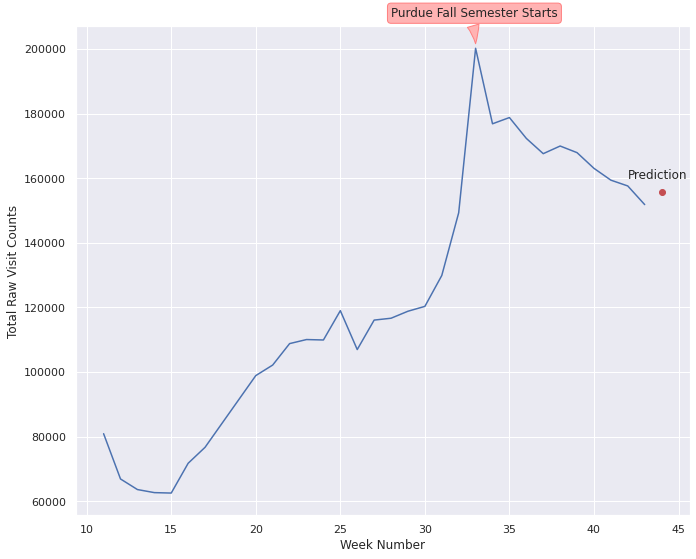# A Closer Look¶

Now let's take a close look at the trend for different variabls when splited into different groups

### Trend of Visits by Place Category (Top 12 categories)¶

The graph below shows the total number of visits by top 10 poi_cbg through the weeks. From the graph we can see that there is a huge spike in some of the categories from week 30 to 33 and a decline after week 33. This is caused by the startinf of Purdue's fall semester.

In :
df2 = df.groupby('poi_cbg')['raw_visit_counts'].sum().sort_values(ascending=False)
# df1 = df1.reset_index()
df2 = df2.iloc[:10]
top_cbg = df[df['poi_cbg'].isin(df2.index)]
p = top_cbg.groupby(['poi_cbg', 'week_number'])['raw_visit_counts'].sum().unstack('poi_cbg').plot(figsize=(20, 10), subplots = True, layout=(5,2))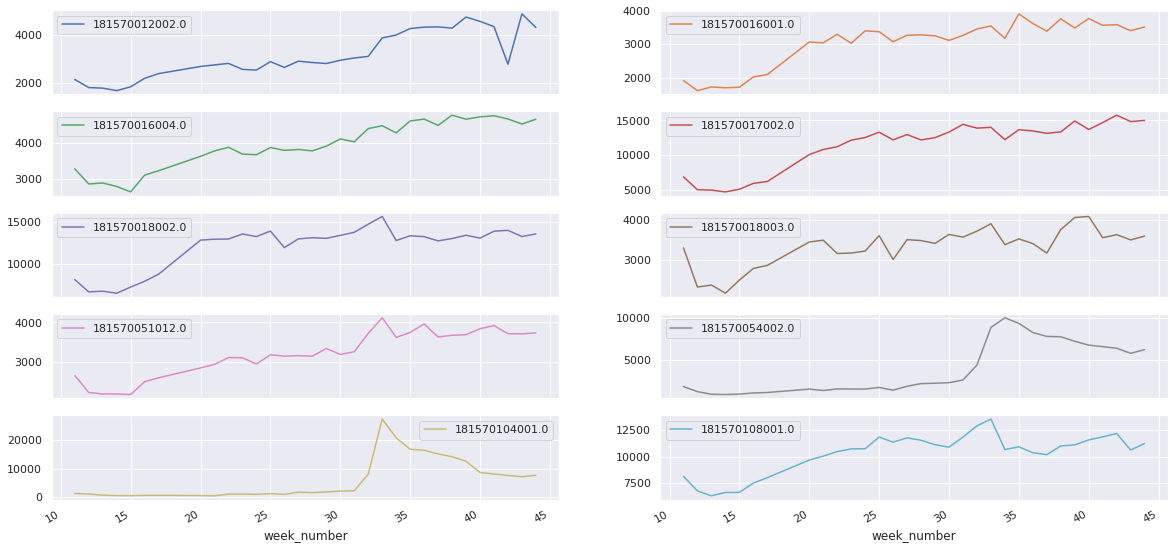### Trend of Visits by Place Category (Top 12 categories)¶

The graph below shows the total number of visits by top 12 categories through the weeks. From the graph we can see that there is a huge spike in some of the categories from week 30 to 33 and a decline after week 33. This is caused by the startinf of Purdue's fall semester. Essential categories such as automotive, health, physicials, dentists are not affected by Purdue's semester start.

In :
df1 = df.groupby('top_category')['top_category'].count().sort_values(ascending=False)
# df1 = df1.reset_index()
df1 = df1.iloc[:12]
cat = df[df['top_category'].isin(df1.index)]
c = cat.groupby(['top_category', 'week_number'])['raw_visit_counts'].sum().unstack('top_category').plot(figsize=(20, 10), subplots = True, layout=(3,4))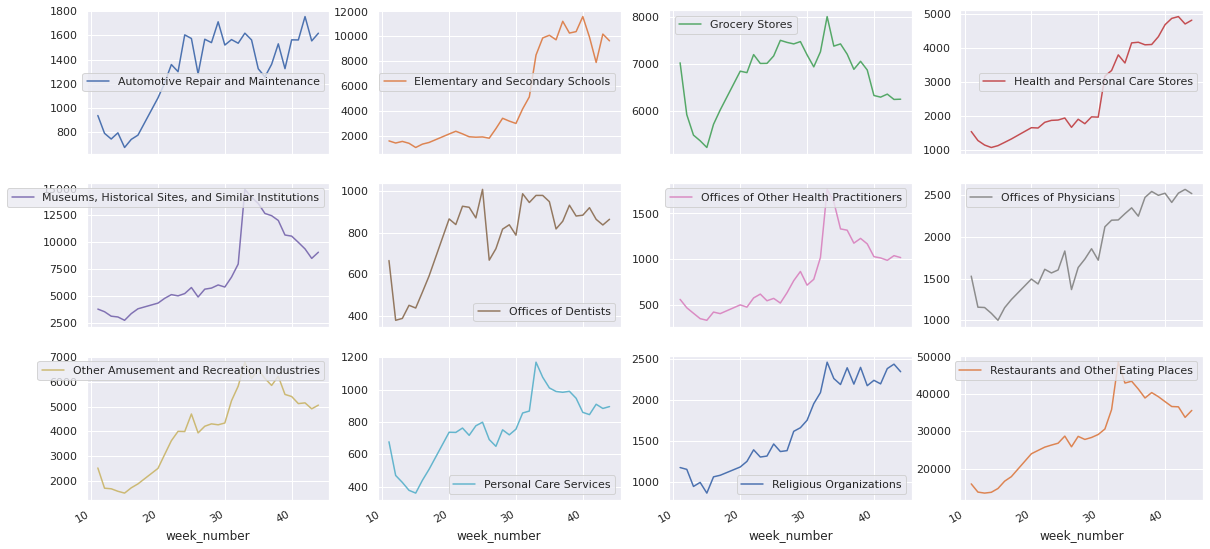### Trend of Visits Concentrations by Place Category (Top 12 categories)¶

The graph below shows the total number of visits concentrations splited by top 12 categories through the weeks. Form this, we can see that visit concentrations for categories such as elementary schools, physicians, religious organizations, restaurants, and recreation industries has been decreasing due to the covid.

In :
p = cat.groupby(['top_category', 'week_number'])['visits_concentration'].sum().unstack('top_category').plot(figsize=(20, 10), subplots = True, layout=(3,4))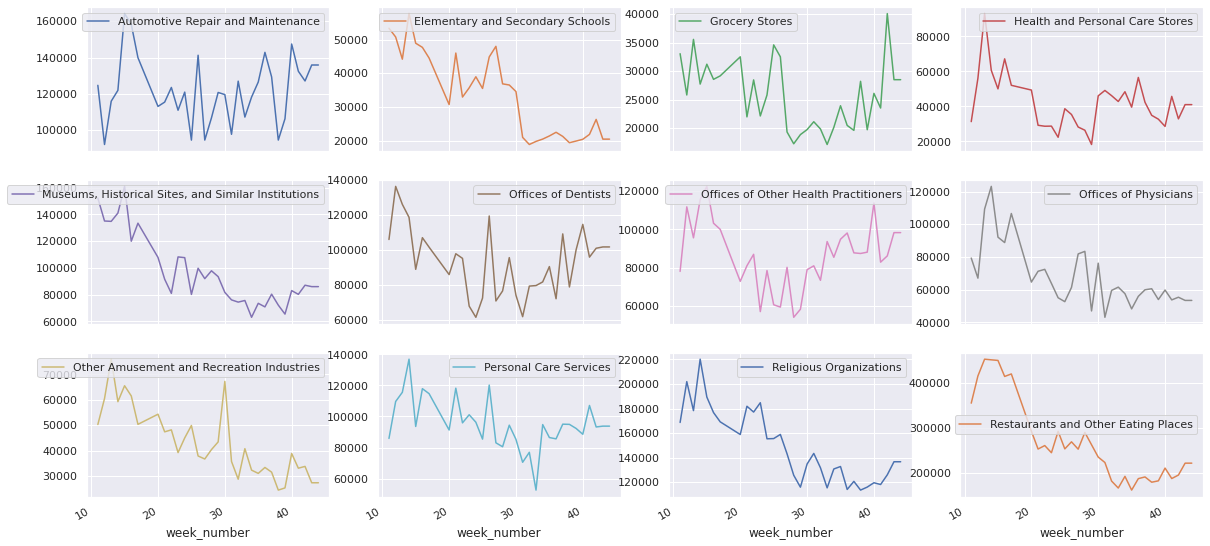### Trend of Visits by Postal Code¶

These graphs below shows the totoal visits splited by postal codes. We can see that different postal codes have drastically different pattern for number of visitors througout the period. We can tell that the postal codes 47906 and 47907 are where Purdue is judging from their trend.

In :
p = df.groupby(['postal_code', 'week_number'])['raw_visit_counts'].sum().unstack('postal_code').plot(figsize=(20, 10), subplots = True, layout=(5,4))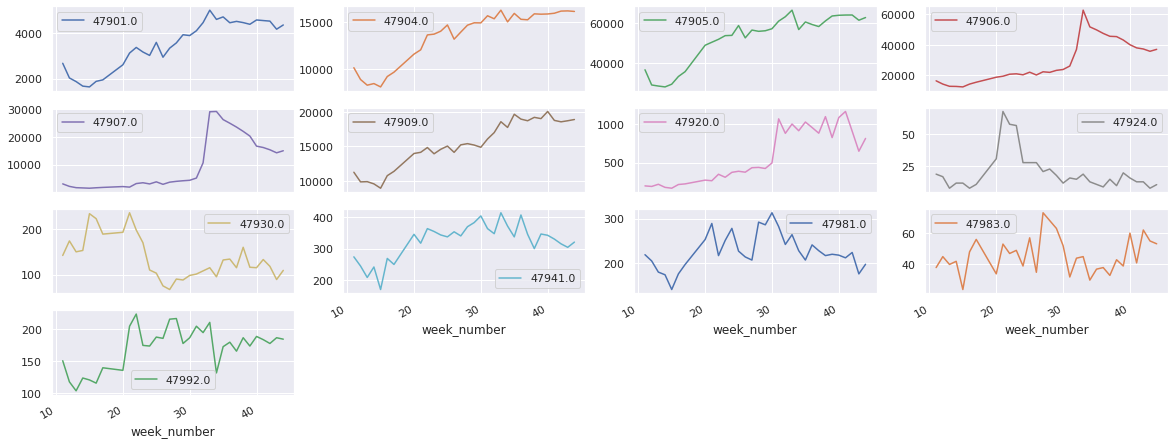### Trend of Visits Concentrations by Postal Code¶

These graphs below shows the visits concentrations splited by postal codes. We can see that different postal codes have drastically different pattern for visits concentrations througout the period.

In :
p = df.groupby(['postal_code', 'week_number'])['visits_concentration'].sum().unstack('postal_code').plot(figsize=(20, 10), subplots = True, layout=(5,4))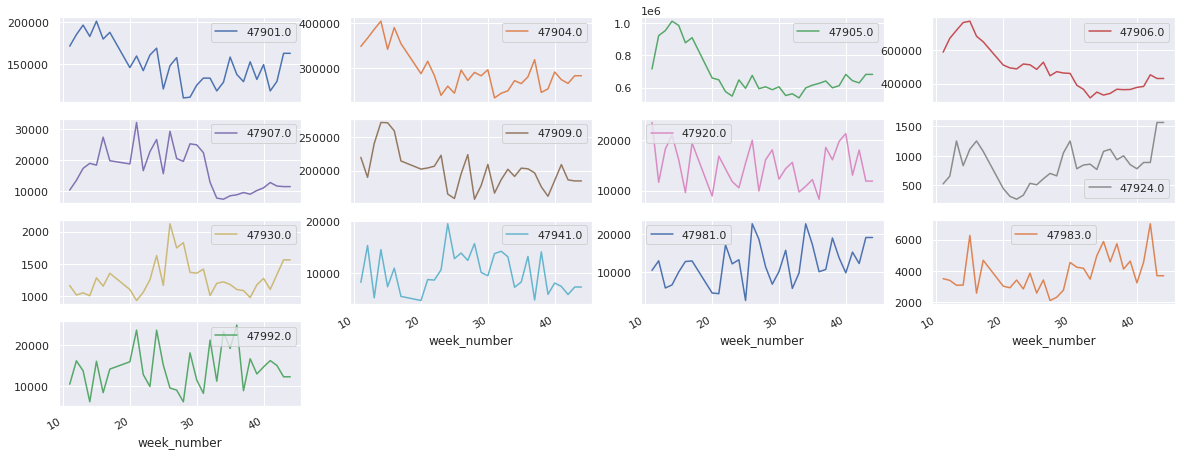In :
df1 = df.groupby('top_category')['top_category'].count().sort_values(ascending=False)
df1 = df1.iloc[:5]
top_cat = df[df['top_category'].isin(df1.index)]
df.loc[~df["top_category"].isin(top_cat['top_category']), "top_category"] = "Others"
wk44 = df[df['week_number']==44]
wk = df[df['week_number'].isin([14,24,34,44])]
wk44['top_category']=wk44['top_category'].replace('Restaurants and Other Eating Places', 'Restaurants').replace('Museums, Historical Sites, and Similar Institutions', 'Museums').replace('Religious Organizations', 'Religious').replace('Automotive Repair and Maintenance', 'Auto Repair').replace('Other Amusement and Recreation Industries', 'Recreation')
top = ('Restaurants', 'Museums','Religious','Auto Repair', 'Recreation','Others')


# Places on the Map¶

### Location on Map with Visit Counts and Top Category for Week 44¶

For the graph below, I ploted the places with matching latitude and longitde for week 44. The different colors represent different categories and the sizes represents the number of visitores for that week. Since the difference between the visitors for places are very big, I averaged them out abit so that the graph shows as much place as possible.

In :
figure = sns.scatterplot('longitude', 'latitude', data=wk44, size ="raw_visit_counts", sizes=(30, 1000), hue="top_category", hue_order = top)

sns.set(rc={'figure.figsize':(11,9)})
plt.show()